# Power combiner loss calculation

New for August 2021. How many times have you been to a design review and see plots of S-parameters of power dividers where the presenter uses hand puppets to describe the loss of the component? Particularly in quadrature couplers, where coupled and through ports are not equal in magnitude, how do you know what the actual loss is? If you averaged the dB losses you would be wrong... if you averaged the S-parameter magnitudes (which are in units of voltage) you'd also be wrong.  You need to add up the square of the S-parameters to ports that you are delivering power to.  In the case of an eight-way corporate Wilkinson, the loss in dB would be:

Eightway_combiner_loss=10*log10(S21M^2+S31M^2+S41M^2+S51M^2+S61M^2+S71M^2+S81M^2+S91M^2)

Good luck measuring that eight-way combiner!

## Example: quadrature 3-dB hybrid coupler

In this example, we downloaded S-parameters for Marki's QH-0R518 0.5 to 18GHz hybrid coupler.  It is a stripline design, and no-doubt a multisection coupler to get that bandwidth.  As a matter of fact, bandwidth like this is only available in pure transverse-electromagnetic (TEM) structures like stripline and coax, microstrip falls down on the job for many reasons. We'd like to thank Marki for providing four-port S-parameters for all of their coupler products!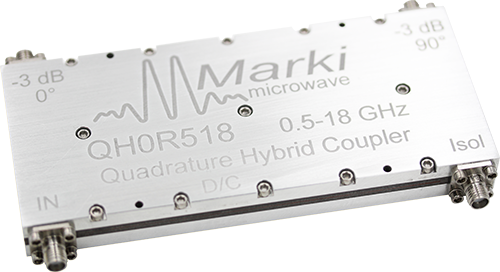First, let's plot the S-parameter magnitudes references to port 1 (it is a symmetric design, so you will get the same results for all ports).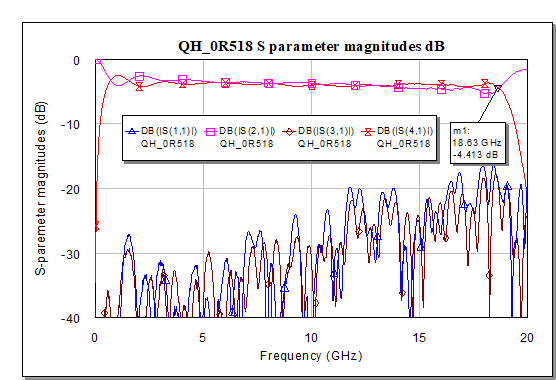One way to project the loss is to look for "kiss points", where the coupled and through paths provide the same transmission. In this example we see them kiss at 18.25 GHz, with loss of -4.413 dB (look for the marker). Subtracting the ideal power split of 3.01 dB, you could say the loss is 1.4 dB at 18.25 GHz and you'd be 100% correct. But what about at other frequencies?

Before we do a bunch of math, we like to make the project generic, so we loaded the Marki data into a four-port circuit we called "combiner test": That way you can download the project and put your own coupler into it easily.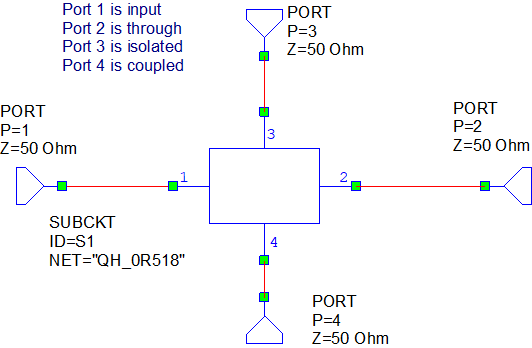Before we get to the loss calculations, let's see how ideal the coupler is regarding phase angle between through and coupled ports.  We put the following equations into the project, subtracting S21 angle from S41 angle. For some reason, MWO equations think S-parameter angles are in radians, so you need to use the "deg" fucntion here: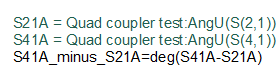Now check out the plot of phase angle below.  It is near perfect. Some of the noise on the data is probably measurement error.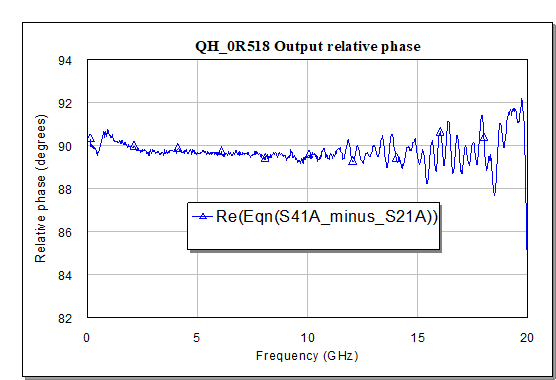Now, let's grab the four S-parameter magnitudes in the equation block and do some math.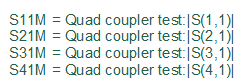As we noted before, you need to square the S-parameters to put the into power units. The efficiency of the coupler is then the sum of powers out the coupled and through ports.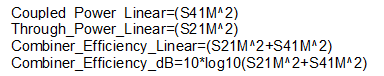Here is a plot of power coming out the coupled and through ports, which adds up to the combiner efficiency, which is 73.3% at 18.25 GHz. Basically, you lose about 1/4 of your available power. Where does the missing power go?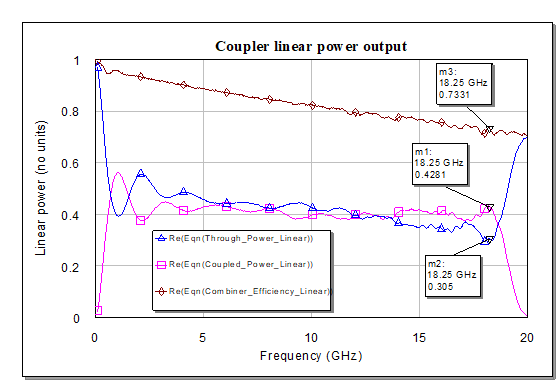Let's look at the loss in dB. Marki advertises it at maxium 1.5 dB loss up to 18 GHz. That is spot-on with our calculation, and if you averaged out the noise you might say the loss is ~1.4 dB.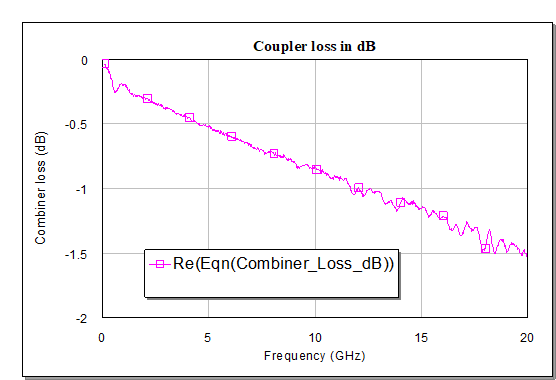Let's consider mismatch loss and isolation loss (power lost out the isolation terminal).  Going back to the orginal plot, S11 and S31 are mostly better than -20 dB, which means not much power is lost out these two ports. Again, you need to square the S-parameters to get units of power.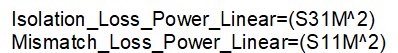In the plot below, at 18.25 GHz, mismatch loss is 0.74%, while isolation loss is remarkable, at just 0.01%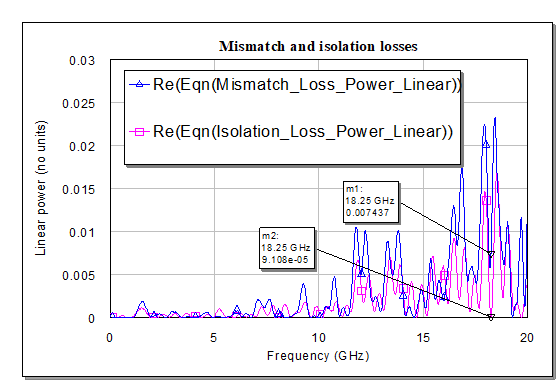Let's calculate dissipative loss. This loss could include radiation loss, there is no way to tell from the data. However, unless you do something really stoopid the majority of the loss in stripline is probably dissipation. In this dissipation loss equation, you need to subtract coupled and through losses from unity, but add back in the mismatch and isolation losses so you are not double-dipping them. If you think about this too much you will either get a headache or need a beer. We chose the latter path.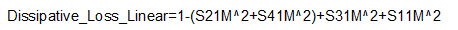Here's the dissipative loss, 25.9% at 18.25 GHz.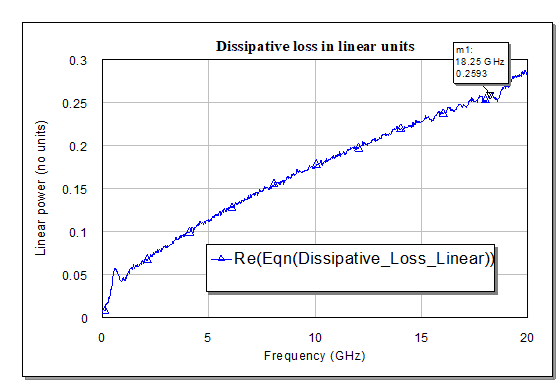Adding together dissipation loss, mismatch loss, isolation loss and combiner efficiency, you should get back account for all energy in the system as a "check-sum" which should equaly unity:

0.2593+0.007437+0.00009108+0.7331=0.99992808

The error is attributed to Microwave Office rounding off the numbers.

We put this Microwave Office project in our download area, if you want to try it on your own quadrature hybrid coupler. You'll need four-port S-parameters, which are not always available from manufacturers.  Thanks to Marki for the example on this page!

Author : Unknown Editor